Next: Cold-Plasma Dielectric Permittivity Up: Waves in Cold Plasmas Previous: Introduction

# Plane Waves in a Homogeneous Plasma

The propagation of small amplitude waves is described by linearized equations. These are obtained by expanding the equations of motion in powers of the wave amplitude, and neglecting terms of order higher than unity. In the following, we use the subscript 0 to distinguish equilibrium quantities from perturbed quantities, for which we retain the previous notation.

Consider a homogeneous, quasi-neutral plasma, consisting of equal numbers of electrons and ions, in which both plasma species are at rest. It follows that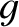, and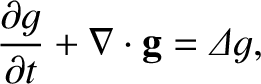. In a homogeneous medium, the general solution of a system of linear equations can be constructed as a superposition of plane wave solutions: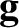(450)

with similar expressions for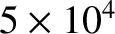and. The surfaces of constant phase,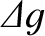(451)

are planes perpendicular to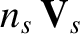, traveling at the velocity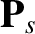(452)

where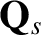, and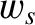is a unit vector pointing in the direction of. Here,is termed the phase-velocity. Henceforth, we shall omit the subscriptfrom field variables, for ease of notation.

Substitution of the plane wave solution (450) into Maxwell's equations yields: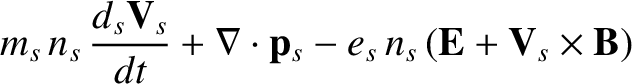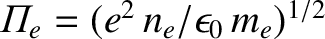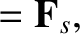(453)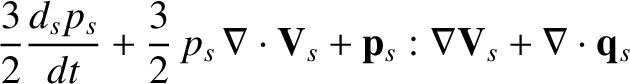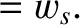(454)

In linear theory, the current is related to the electric field via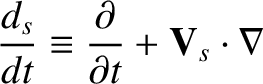(455)

where the conductivity tensor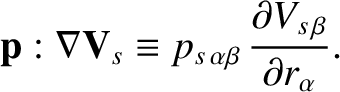is a function of bothand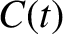. Note that the conductivity tensor is anisotropic in the presence of a non-zero equilibrium magnetic field. Furthermore,completely specifies the plasma response.

Substitution of Eq. (455) into Eq. (453) yields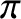(456)

where we have introduced the dielectric permittivity tensor,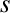(457)

Here,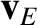is the identity tensor. Eliminating the magnetic field between Eqs. (454) and (456), we obtain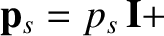(458)

where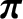(459)

The solubility condition for Eq. (459),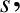(460)

is called the dispersion relation. The dispersion relation relates the frequency,, to the wave-vector,. Also, as the name dispersion relation'' indicates, it allows us to determine the rate at which the different Fourier components in a wave-train disperse due to the variation of their phase-velocity with wave-length.Next: Cold-Plasma Dielectric Permittivity Up: Waves in Cold Plasmas Previous: Introduction
Richard Fitzpatrick 2011-03-31